Question

I know that the answer is a but what are the steps?? Q12. A stone is...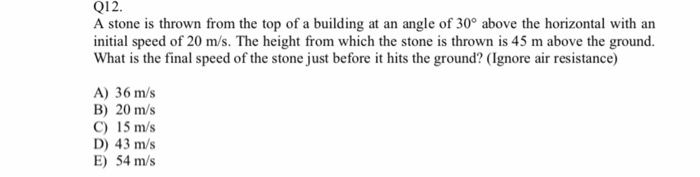I know that the answer is a but what are the steps??
Q12. A stone is thrown from the top of a building at an angle of 30° above the horizontal with an initial speed of 20 m/s. The height from which the stone is thrown is 45 m above the ground. What is the final speed of the stone just before it hits the ground? (Ignore air resistance) A) 36 m/s B) 20 m/s C) 15 m/s D) 43 m/s E) 54 m/s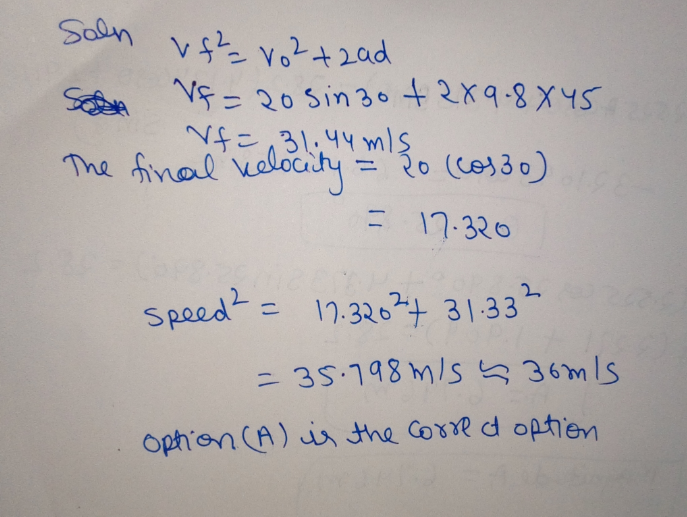Earn Coins

Coins can be redeemed for fabulous gifts.

Similar Homework Help Questions
• A stone is thrown with an initial speed of 10 m/s at an angle of 32...

A stone is thrown with an initial speed of 10 m/s at an angle of 32 degrees above the horizontal from the top of a 35 m building. If air resistance is negligible, then what is the speed of the rock as it hits the ground?

• A stone thrown horizontally from the top of a 22-m tower hits the ground at a...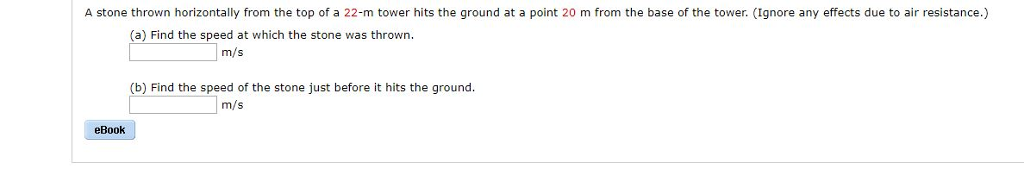A stone thrown horizontally from the top of a 22-m tower hits the ground at a point 20 m from the base of the tower. (Ignore any effects due to air resistance.) (a) Find the speed at which the stone was thrown m/s (b) Find the speed of the stone just before it hits the ground m/s eBook

• can you draw a coordinate system as well please and show all steps. Thank you A...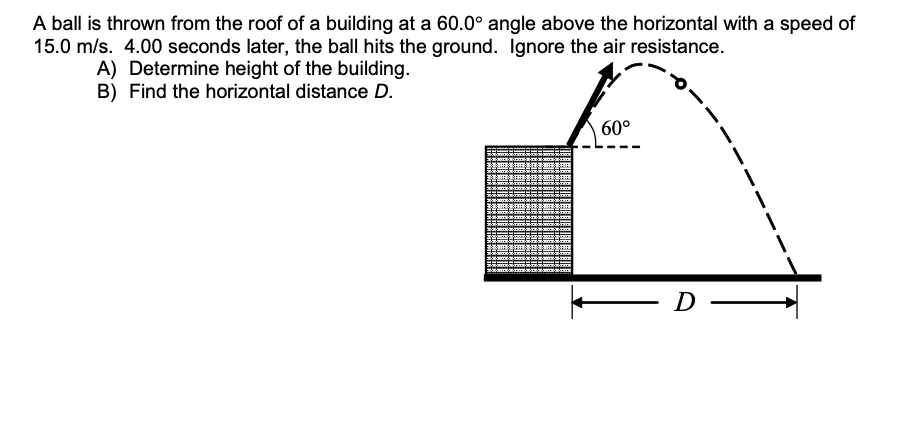can you draw a coordinate system as well please and show all steps. Thank you A ball is thrown from the roof of a building at a 60.0° angle above the horizontal with a speed of 15.0 m/s. 4.00 seconds later, the ball hits the ground. Ignore the air resistance. A) Determine height of the building. B) Find the horizontal distance D. 60° 1 D

• A stone is thrown from the top of a building at an angle of 30 degrees to the horizontal and with an initial speed of 20 m/s

A stone is thrown from the top of a building at an angle of 30 degrees to the horizontal and with an initial speed of 20 m/s. If the height of the building is 45 m, find a) the time it takes for the stone to reach the ground, b) the distance between the object and the building.**I need to know step by step how to solve it. The teacher already went over it and I get the basics but...

• A stone thrown horizontally from the top of a 24-m tower hits the ground at a...

A stone thrown horizontally from the top of a 24-m tower hits the ground at a point 18 m from the base of the tower. (Ignore any effects due to air resistance.) (a) Find the speed at which the stone was thrown. (b) Find the speed of the stone just before it hits the ground.

• Thrown stone

A stone thrown horizontally from a height of 6.17 m hits the ground at a distance of 12.30 m. Calculate the initial speed of the stone. Neglect air resistance.Calculate the speed of the stone as it hits the ground. Neglect air resistance.

• A stone thrown horizontally from a height of 6.27 m hits the ground at a distance...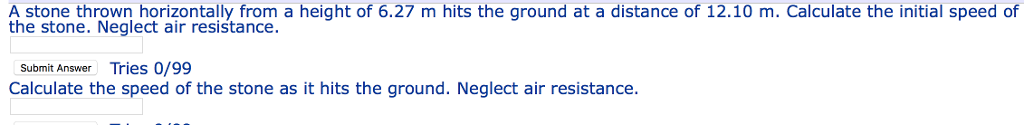A stone thrown horizontally from a height of 6.27 m hits the ground at a distance of 12.10 m. Calculate the initial speed of the stone. Neglect air resistance. Submit Answer Tries 0/99 Calculate the speed of the stone as it hits the ground. Neglect air resistance.

• I know that the answer is a but what are the steps ?? A rock is...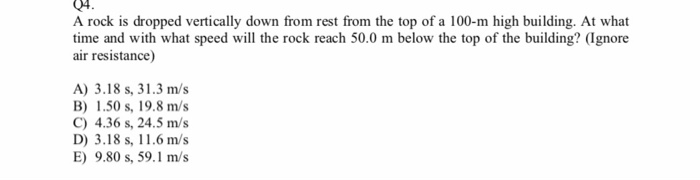I know that the answer is a but what are the steps ?? A rock is dropped vertically down from rest from the top of a 100-m high building. At what time and with what speed will the rock reach 50.0 m below the top of the building? (Ignore air resistance) A) 3.18 s, 31.3 m/s B 1.50 s, 19.8 m/s C) 4.36 s, 24.5 m/s D) 3.18 s, 11.6 m/s E) 9.80 s, 59.1 m/s

• Motion in a Plane with constant Acceleration

From the edge of a rooftop of the building, a boy throws a stone at an angle of 25.0 degrees above the horizontal. The stone hits the ground 4.20s later, 105 maway from the base of the building. (Ignore air resistance). (a) for the stones path through the air, sketch graphs of x, y, Vx, and Vy as functions of time. Theseneed to be only qualitatively correct-- you need not put numbers on the axes. (b) Find the initial velocity...

• A stone is thrown horizontally from the top of a 31 m building. The stone hits...

A stone is thrown horizontally from the top of a 31 m building. The stone hits the ground at a point 19 m from the base of the tower. 1. Determine the speed with which the stone is thrown. 2. Determine the speed of the stone just before it hits the ground. Explain Please!!